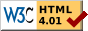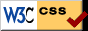The Meaning of Planck’s Constant Jan12, 2008 Note: For the benefit of those who might not have read the previous articles, the foundational basis of Zeron Theory is repeated at the beginning of each article. THE MEANING OF PLANCK’S CONSTANT AND DERIVATION OF THE MASS OF A ZERON This article reveals one of the most enduring mysteries in physics, the meaning of Planck’s Constant. It also shows the derivation of the mass of the elusive Zeron. The quantification of this mass adds considerable weight to the veracity of Zeron theory. In this very short article I need to start with some models that were more rigorously derived in the original development of the theory. You can get more information by following the link at the end of this article. The Basic Foundational Model ·There is an Aether. It consists of an all-pervasive ’atmosphere’ of prime particles called Zerons ·All Zerons are identical, and are totally elastic, frictionless, have inertial mass but zero gravitational mass, and come in two varieties, fast and slow. ·Fast Zerons travel at a velocity of root 2 x c and inter-collide to form a type of Aether which we have named the Cosma. The Cosma is a resonant Perfect Fluid. ·Matter consists of resonating agglomerations of Slow Zerons held together by pressure from the Cosma. ·Matter resonates at a frequency different to that of the Cosma. ·The Zeron constitutes an unimaginably small class of matter and can be shown to have a mass of 7.39 x10p-46 gms compared to an electron mass of 9.11 x 10p-28 gms. There are therefore 1, 33 x 10p18 Zerons in an electron! The Basic Foundational Atom Consider the hydrogen atom. The Proton nucleus consists of an agglomeration of slow resonating Zerons within the resonant Cosma. Their interaction produces a denser region in the Cosma in the form of a spherical standing wave shell of Zerons. This is the electron shell. Light emanates from the atom after it is first excited so that the electron shell jumps to the next permissible radius and then collapses to emit an outwardly expanding sphere of light manifested as a series of Zeron impact waves travelling through the Cosma at a velocity c in every direction. If we now accelerate the atom through the Aether the Zeron pressure at the front of the nucleus becomes greater than at the back. This distorts both the nucleus and the electron shell. As soon as the acceleration stops a steady state returns and the atom retains its distorted shape. Planck’s puzzling constant h, derived from the graph of black body radiation, and one of the foundational constants of Quantum Physics, has always been thought of as some sort of manifestation of the underlying "grain" of matter. The constant is exceedingly small, and has the strange dimension of erg-sec. It comes into play in virtually all quantum calculations. What this constant (or its companion h/2pi) represents, if anything, has always been a mystery. Zeron theory provides a rational explanation. We have seen that light emanates from an atom as the Zeron electron shell collapses from a stable allowable radius to the next smaller allowable radius. In the process it sends out an expanding shell of Zeron momentum energy that is the matrix from which the ’’photon’’ is formed. The photon consists of a series of Zeron impact waves and contains some 10p5 to 10p6 oscillations. One equation that incorporates Planck’s constant is for the energy of a photon. E = h x f ..................................... (1) Where E is the energy of a photon, h = Planck’s constant and f is the frequency of the photon. The Zeron interpretation of the photon is that while, in a general sense, it is not the Zerons that are translated by the light wave but rather it is the Zeron momentum that is translated through the Cosma, there is, for each light wave oscillation, the movement of Zerons by one "pitch". The foremost Zeron in the path of the photon wave dumps its energy on the absorbing surface by impacting on the surface. It loses its kinetic energy through absorption into the electron shell of the atoms on the surface. The total energy of the "dumped" Zerons is the sum of the kinetic energies of all the Zerons. Now the total number of Zerons dumped is the frequency of the light packet times the period for which it is transmitted, i.e. f x secs. Going back to Newton, the total kinetic energy of the Zerons can be expressed as E = 1/2 Mz x Vz x Vz x f x secs..................................(2) Where Mz is the Zeron mass and Vz is its velocity. But the velocity of the Zeron is root2 x c where c = the velocity of light. The energy dumped by the light train is therefore E = 1/2 x Mz x root 2 c x root 2 c x f x secs = Mz c2 x f x secs..... (3) We have therefore two equations (1) and (3) for the energy of a photon. Equating them gives h x f = Mz c2 x f x secs Dividing both sides by f h = Mz c2 secs But the dimensions of h are erg.secs and if we measure the photon energy in ergs, we get: h (erg secs) = Mz c2 (erg secs) Dividing both sides by erg x secs h = Mz c2 ................................................ (4) Does this look familiar? Compare this with E = M c2 Planck’s Constant h turns out to be the energy of a fast-Zeron. Planck’s Constant is indeed a measure of the underlying grain of matter. It was just that nobody knew what sort of grain. Equation (4) is the quantum equivalent of the macro expression for Einstein’s equation for the equivalence of mass and energy. Here once more we see the melding of macro Physics (Relativity etc) and micro physics (Quantum mechanics). It’s a most satisfactory outcome. It seemed highly unlikely that one could derive the mass of an undetectable particle, but that is exactly what we are about to do. From equation (4) above, we have Mz = h/c2 Substituting experimental values for c and h we get Mz = 6.63 x 10 p-27 / 2.995x2.995 x 10p10 x 10p10 Zeron mass = Mz = 7.39 x 10p -46 gms ! This is an exceedingly small mass. Even the tiny electron is composed of a great number of Zerons namely 9.11 x 10p-28 / 7.39 x 10p-46 1 Electron mass = 1.33 x 10p18 Zeron masses! While it was always clear that in order for the Zeron to produce "Zeron pressure" on a nucleus, it would have to be many orders of magnitude smaller than the nucleus, the incredible minuteness of the Zeron still came as a bit of a surprise. I now know how the early physicists felt when they discovered that the atom only had a very small speck of matter at its center and was seemingly mostly empty space. To drive the point home there are 1,330,000,000,000,000,000 Zerons in an electron! No wonder the Zeron is unlikely to be detected. The unimaginable minuteness of the Zeron lends great credence to the mechanics of the theories so far put forward, especially that of the ability of the Cosma to exert pressure on the atomic nucleus. At the same time the Zeron represents a previously unknown and unimagined class of matter whose size is difficult to comprehend. In rough terms, the Zeron is to the electron as the electron is to a tennis ball!! The dimensions of the Zeron, its velocity, and its physical properties make it clear why the Zeron will probably never be subject to direct detection by instruments which in themselves are composed of the enormously larger atoms. Incredible as it seems, we appear to exist in a Zeron "soup" which we are totally unable to detect! This soup is a different sort of Higgs Field, the postulated all-pervading field consisting of Higgs Bosons. The conventional scientists were right in the postulate but wrong in their assumptions about the sort of field. Our field is the Cosma and our Particle is the Zeron. If this article has piqued your interest you could go to www.tedjaeckel.com http://www.tedjaeckel.com for more information. In Article 7 I will be describing why Uncertainty Theory is invalid and make the case for a return to Newtonian based physics. I hope I have not trodden on too many TOEs. Critique of what is presented here is always welcome. Ted Jaeckel (Tedjay) ```~These Links are a Direct connection to all Institute Documents~ About the Institute  Constitution  Ecumenity  What is Non-Theory?  What is Anti-Theory?   Curriculum  Practical Non-Theory Vote  Efficiency of Machines  Distribution and Production  Commercial Exchange   Oxymoron’s and Other Papers  What about Kyoto?  Rant about Garbage  Global Handbook  Mainstream Science   Remember Hippies?  Free Thinking  Optimism  Add Your Effort  David Suzuki Site``` ```~These Feature Galleries are all RealRufus Kidsafe Areas~ The Gang  The Institute  Written Articles  Froggy & Cats  Eye Puzzlez  Web Design and The Old Page Ruby’s Creative Arts  Ruby’s Garden  Ceramics  Decorator~Designs~Vessels  Studio  Kayaks & Trimaran Norm’s Custom Shop  Digital Photo Gallery  Garage-Studio  Bicycles  Lotus  Motorcycles  Zdravko Z28   Email is to webmaster nsoar@tbaytel.net```